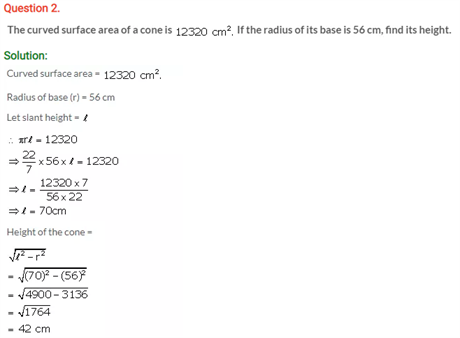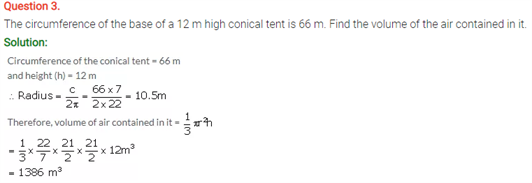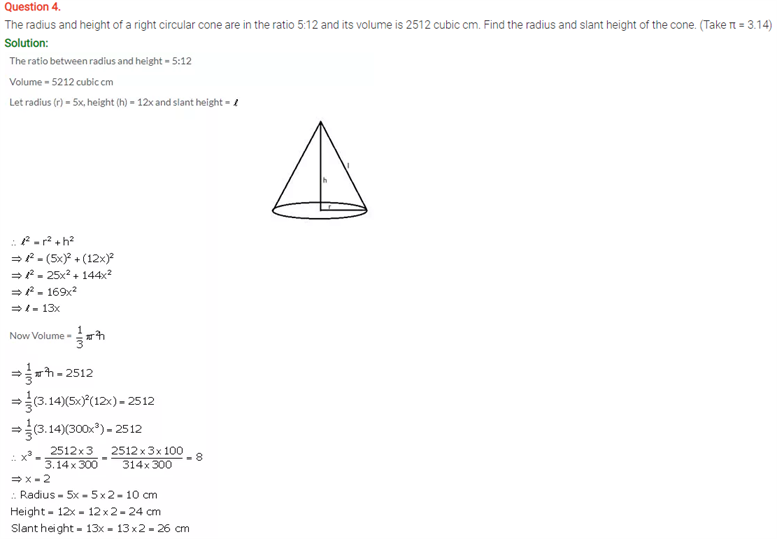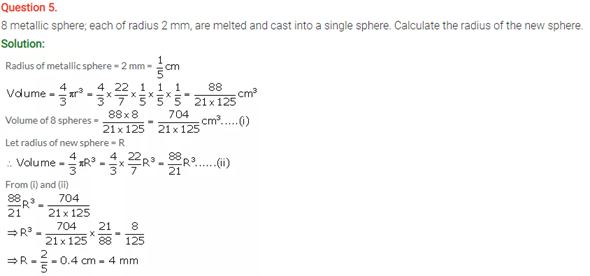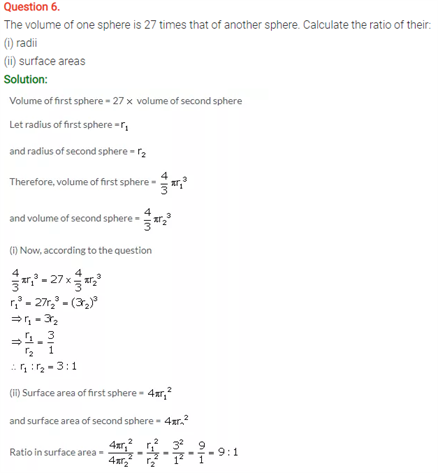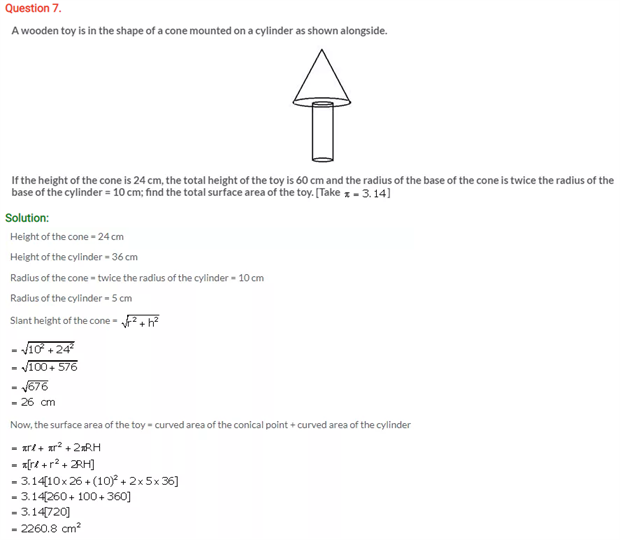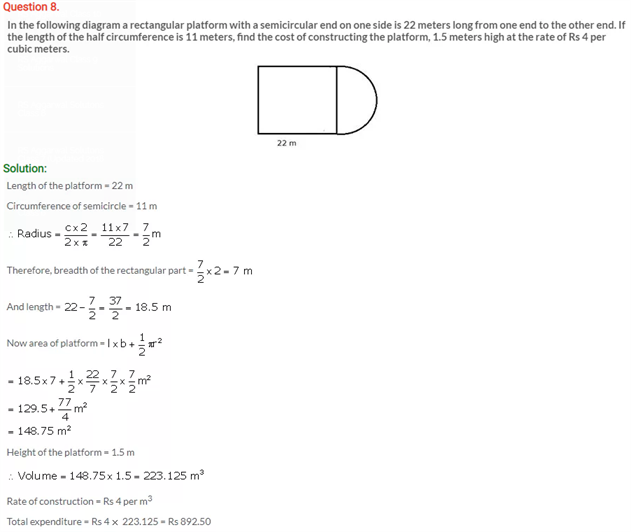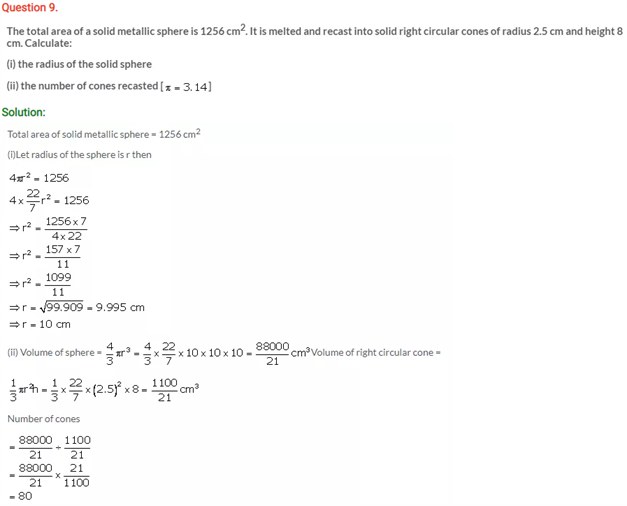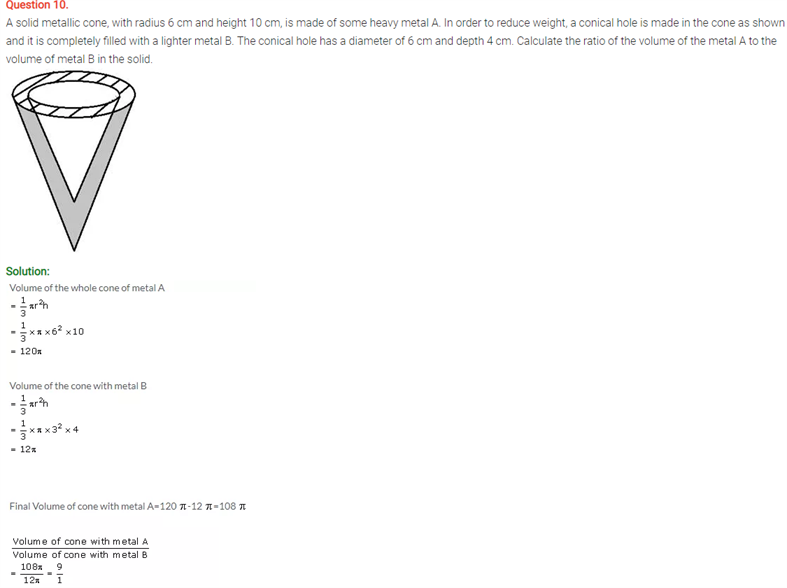# Selina Concise Maths Solution for ICSE Class 10 Chapter 20 Cylinder, Cone and Sphere

Solving the solutions on the cylinder, cone and sphere from the ICSE Selina Solutions For Class 10 Maths will give you clarity on this chapter. In a cylinder, the height h is the perpendicular distance between the bases. It is important to use the perpendicular height (or ‘altitude’) when calculating the volume of an oblique cylinder. The radius r of a cylinder is the radius of a base. If you are given the diameter instead, remember to halve it. An axis is a line joining the center of each base. A cone is a solid that has a circular base and a single vertex. If the vertex is over the center of the base, it is called a right cone. If it is not, it is called an oblique cone. An object that is shaped like a cone is said to be ‘conical’. These questions below belong to the above-mentioned concepts.

Check out the Class 10 Selina Maths ICSE Solutions Chapter 20 Cylinder, Cone and Sphere below: